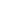C.M.S. • Fredericton, NB • June 2010
Meditations on Arens MultiplicationF.E.J. Linton • Wesleyan Univ. Math/CS Emeritus
Write T for the usual (compact, T2) circle group, C = |T| for its underlying (discrete) Abelian group. The Bohr compactification βA of discrete Abelian group A “is” the left adjoint to | |: AbGp(KT2)AbGp. Its (discrete Abelian) character group (βA)^ is AbGp(KT2)A, T) ~ AbGp(A, |T|) = AbGp(A, C) = A*. And it itself is (Pontryagin) the (compact, Abelian) character group of that: βA = (A*)^. Thus A** = |(A*)^| = |βA|.
Parallels with the Ban1 situation:
X* = underlying Banach space of **-algebra (iX)*: X*** → X*
“=” Banach space X* with compact (weak X)-topology on its unit disk, just as